#### You may also like### Pent

The diagram shows a regular pentagon with sides of unit length. Find all the angles in the diagram. Prove that the quadrilateral shown in red is a rhombus.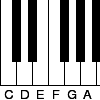### Tuning and Ratio

Why is the modern piano tuned using an equal tempered scale and what has this got to do with logarithms?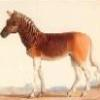### Rarity

Show that it is rare for a ratio of ratios to be rational.

# Another Triangle in a Triangle

### Why do this problem?

This problem offers students the opportunity to discover and prove a result about triangles divided into smaller areas. We hope there will be a moment of surprise leading to curiosity as students desire to explain the result. Students might tackle the problem using ratios and similar triangles, or they might use it to practise manipulating vectors.

This would be a good problem for students to explore using dynamic geometry software such as GeoGebra.

### Possible approach

Students may benefit from working on Triangle in a Triangle and Areas and Ratios before tackling this problem.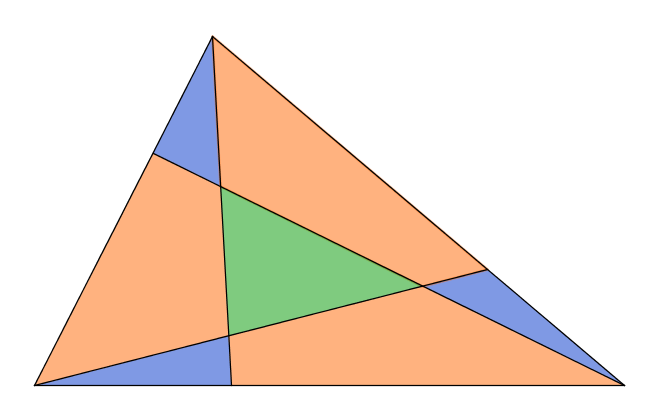Begin by sharing the diagram above and explaining to students how it was created - each line joins a vertex to a point $\frac13$ of the way along the opposite side. Ask students to guess what fraction of the area is shaded green - they may wish to suggest a lower and upper bound rather than guessing an exact amount.

Then invite them to consider ways of finding the exact area.

Below are the series of hints from the problem, which you could offer to students as they are working.

The base of triangle $a+f+e$ is $\tfrac{1}{3}$ of the base of the whole triangle.

Can you use this to work out the area of $a+f+e$ as a fraction of the whole triangle?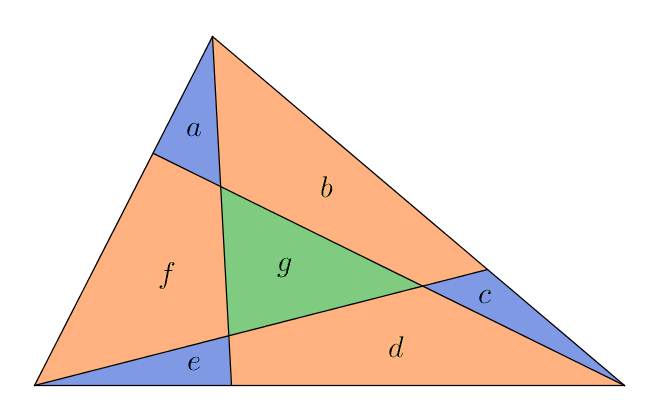What about $a+b+c$ and $c+d+e$?

We have three triangles, each with an area of $\tfrac{1}{3}$ of the whole triangle.

But none of these tell us anything about $g$.

But we do know that $a$, $b$, $c$, $d$, $e$, $f$ and $g$ together make the whole triangle!

Can we combine all this to find an expression for $g$ in terms of other areas?

It would be useful to know the areas of the little blue triangles...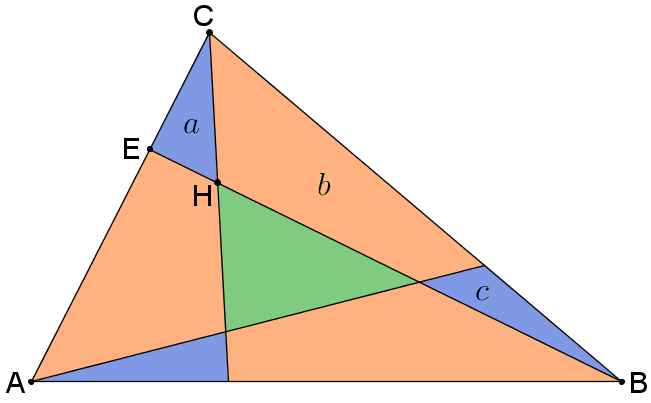We know that:
• $a$ has the same height as triangle $ECB$.
• Triangle $ECB$ is $\tfrac{1}{3}$ of the whole triangle

If we can find the length $EH$ as a fraction of $HB$, we could work out the area of the blue triangle...

Sometimes it helps to add in an extra line...Can you find some similar triangles in this diagram?

How does $EJ$ compare with $AF$?

How does $EJ$ compare with $BF$

How does $EH$ compare with $HB$?

How does $EH$ compare with $EB$?

Alternatively, if you want your students to focus on vector methods, you could share a diagram such as the one below, in which vectors $\bf{x}$ and $\bf{y}$ have been defined such that $\overrightarrow{AC}=3\bf{x}$ and $\overrightarrow{AB}=3\bf{y}$.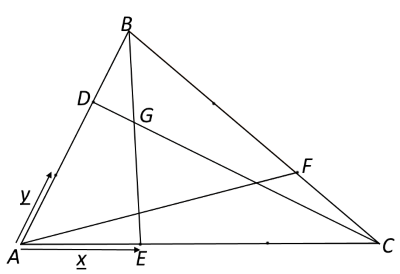You could share these key questions:
Can you express $\overrightarrow{DC}$ in terms of $\bf{x}$ and $\bf{y}$?
Can you express $\overrightarrow{BE}$in terms of $\bf{x}$ and $\bf{y}$?

By writing $\overrightarrow{DG}$ as $\lambda \overrightarrow{DC}$ and $\overrightarrow{BG}$ as $\mu \overrightarrow{BE}$, can you find two expressions for $\overrightarrow{AG}$?

By equating coefficients of $\bf{x}$ and $\bf{y}$, can you find $\lambda$?

### Possible extension

Students could explore what happens if a fraction other than $\frac13$ is chosen to divide the sides of the original triangle.

### Possible support

Triangle in a Triangle is a more accessible problem on a similar theme.